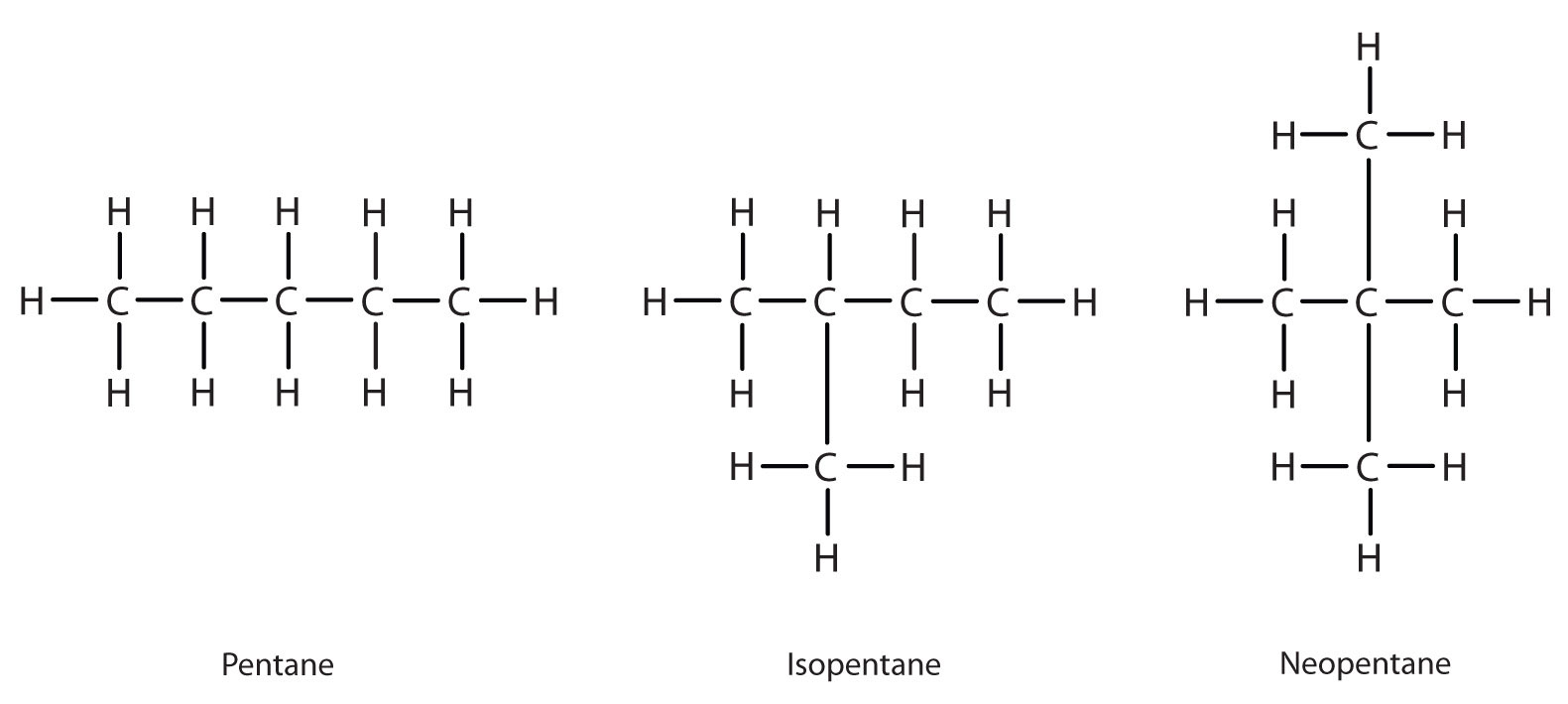Skip to main content

# 11.3: Branched-Chain Alkanes

Learning Objectives

• To learn how alkane molecules can have branched chains and recognize compounds that are isomers.

We can write the structure of butane (C4H10) by stringing four carbon atoms in a row,

–C–C–C–C–

and then adding enough hydrogen atoms to give each carbon atom four bonds:The compound butane has this structure, but there is another way to put 4 carbon atoms and 10 hydrogen atoms together. Place 3 of the carbon atoms in a row and then branch the fourth one off the middle carbon atom:Now we add enough hydrogen atoms to give each carbon four bonds.Figure $$\PageIndex{1}$$).Figure $$\PageIndex{1}$$: Butane and Isobutane. The ball-and-stick models of these two compounds show them to be isomers; both have the molecular formula C4H10.

Notice that C4H10 is depicted with a bent chain in Figure $$\PageIndex{1}$$. The four-carbon chain may be bent in various ways because the groups can rotate freely about the C–C bonds. However, this rotation does not change the identity of the compound. It is important to realize that bending a chain does not change the identity of the compound; all of the following represent the same compound, butane:The structure of isobutane shows a continuous chain of three carbon atoms only, with the fourth attached as a branch off the middle carbon atom of the continuous chain, which is different from the structures of butane (compare the two structures in Figure $$\PageIndex{1}$$.

Unlike C4H10, the compounds methane (CH4), ethane (C2H6), and propane (C3H8) do not exist in isomeric forms because there is only one way to arrange the atoms in each formula so that each carbon atom has four bonds.

Next beyond C4H10 in the homologous series is pentane. Each compound has the same molecular formula: C5H12. (Table 12.1.1 from the previous section has a column identifying the number of possible isomers for the first 10 straight-chain alkanes.) The compound at the far left is pentane because it has all five carbon atoms in a continuous chain. The compound in the middle is isopentane; like isobutane, it has a one CH3 branch off the second carbon atom of the continuous chain. The compound at the far right, discovered after the other two, was named neopentane (from the Greek neos, meaning “new”). Although all three have the same molecular formula, they have different properties, including boiling points: pentane, 36.1°C; isopentane, 27.7°C; and neopentane, 9.5°C.A continuous (unbranched) chain of carbon atoms is often called a straight chain even though the tetrahedral arrangement about each carbon gives it a zigzag shape. Straight-chain alkanes are sometimes called normal alkanes, and their names are given the prefix n-. For example, butane is called n-butane. We will not use that prefix here because it is not a part of the system established by the International Union of Pure and Applied Chemistry.

• Was this article helpful?